/

### The inverse gamma distribution

The inverse gamma distribution describes the distribution of the reciprocal of a variable distributed according to the gamma distribution.

 Distribution name Inverse gamma Common notation Parameters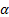= shape parameter (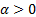)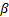= scale parameter (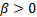) Domain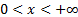Probability density function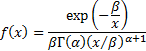Cumulative distribution function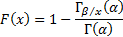Mean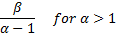Variance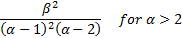Skewness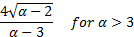(Excess) kurtosis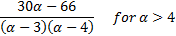Characteristic function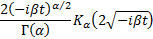Other comments Also called the log Pearson type 5 distribution.   Its mode is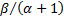Nematrian web functions

Functions relating to the above distribution may be accessed via the Nematrian web function library by using a DistributionName of “inverse gamma”. Functions relating to a generalised version of this distribution including an additional location (i.e. shift) parameter may be accessed by using a DistributionName of “inverse gamma3”, see also including additional shift and scale parameters. For details of other supported probability distributions see here.